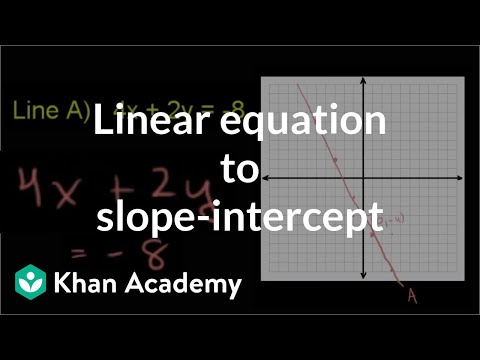Video

# Worked example: intercepts from an equation (Full video)

Khan Academy

## Supporting grades: 8

Description: Sal demonstrates how to find the x and y-intercepts of an equation in order to graph it. We're told to find the x- and y-intercepts for the graph of this equation: 2 y plus 1/3x is equal to 12. And just as a bit of a refresher, the x-intercept is the point on the graph that intersects the x-axis. And by the exact same argument, the y-intercept occurs when we're not to the right or the left of the y-axis, so that's when x is equal to 0.

You must log inorsign upif you want to:*

*Teacher Advisor is 100% free.

### Other videos you might be interested in### Ratio and Proportion in Grades 6-8: Connections to College and Career Skills (Full video)

#### The Hunt Institute